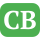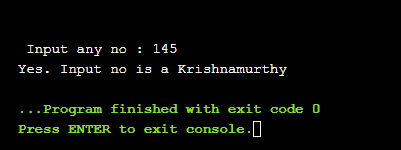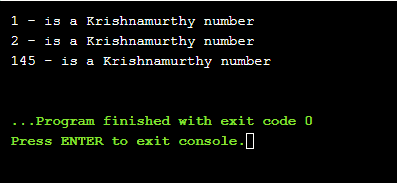CppBuzz.com

# C++ Program to check if number is Krishnamurthy

A Krishnamurthy number is a number whose sum of the factorial of digits is equal to the number itself. For example 145, 1! + 4! + 5! = 1 + (4*3*2*1) + (5*4*4*2*1) = 1 + 24 + 120 = 145. This C++ program takes input from user and check if it is Krishnamurthy. Program is divided into functions factorial(int) and isKrishnamurthy(int). For more details please run this code.

``````#include <iostream>
using namespace std;

//Function to calculate the factorial of any number
int factorial(int n)
{
int fact = 1;
while (n != 0)
{
fact = fact*n;
n--;
}
return fact;
}

//function to Check if number is krishnamurthy
void isKrishnamurthy(int n)
{
int sum = 0;

int temp = n;
while (temp != 0)
{
// calculate factorial of last digit
// of temp and add it to sum

sum += factorial(temp%10);

// replace value of temp by temp/10
temp = temp/10;
}

// Check if number is krishnamurthy

if(sum == n)
cout << "Yes. Input no is a Krishnamurthy";
else
cout << "No. Input no is not a Krishnamurthy";
}

int main()
{
int n;
cout<<"\n Input any no : ";
cin>>n;

isKrishnamurthy(n);

return 0;
}```
```

## 145 is a Krishnamurthy number## Finding Krishnamurthy numbers between 1 & 1000

We will use the above program only, but we will call isKrishnamurthy() function inside the for loop which runs from 1 to 1000

``````#include <iostream>
using namespace std;

//Function to calculate the factorial of any number
int factorial(int n)
{
int fact = 1;
while (n != 0)
{
fact = fact*n;
n--;
}
return fact;
}

//function to Check if number is krishnamurthy
void isKrishnamurthy(int n)
{
int sum = 0;

int temp = n;
while (temp != 0)
{
// calculate factorial of last digit
// of temp and add it to sum

sum += factorial(temp%10);

// replace value of temp by temp/10
temp = temp/10;
}

// Check if number is krishnamurthy

if(sum == n)
cout<<n<<" - is a Krishnamurthy number"<<endl;
}

int main()
{

for(int i = 1; i<1000; i++)
{
isKrishnamurthy(i);
}

return 0;
}

``````

## 1, 2 & 145 are Krishnamurthy numbers